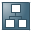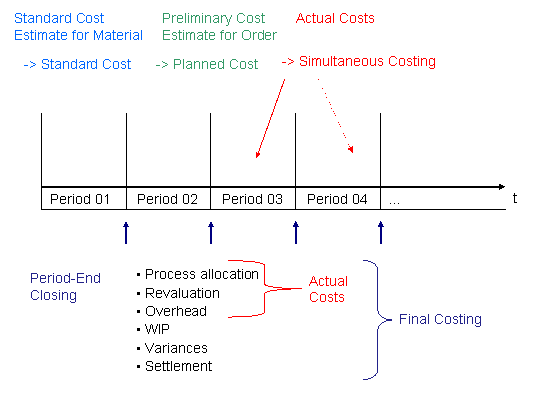Show TOC

###Sequence of Steps in Cost Object Controlling: ScenarioIn the Product Cost Planning component, you first create a standard cost estimate for the material to establish the standard cost.

#### Process

After creating a standard cost estimate, you carry out the following steps in the Cost Object Controlling component:

1. Preliminary costing

Preliminary costing calculates the planned cost for each object. For example, if the cost object is a production order, planned costs can be used in variance calculation as the basis for calculating target costs.

2. Simultaneous costing

All actual costs incurred for a cost object are collected directly on the cost object. Actual costs are incurred in different ways, such as through internal activity allocations and material withdrawals. This process of collecting actual costs on a cost object is called simultaneous costing.

3. Final costing

Once the cost object has been produced, final costing determines the actual costs incurred during the production process. Final costing also serves the purposes of cost analysis and control.

Final costing is normally performed during period-end closing.

The period-end closing process includes the capture of period costs, which are actual costs that are not attributable to the cost object from a particular activity. This can be the revaluation of activities at actual prices or the calculation of process costs or overhead, for example.

The period-end closing process can also determine the value of unfinished goods (work in process).

You can calculate variances in the period-end closing process in Product Cost by Period and Product Cost by Order.

When you settle, you can transfer data to other application components such as Financial Accounting.

Period-end closing in Cost Object Controlling is performed after period-end closing in Cost Center Accounting.

##### Sequence of Steps in Cost Object ControllingIn a make-to-stock environment, you normally create standard cost estimates for your materials in the Product Cost Planning component. These cost estimates calculate the standard costs of the materials. The sum of the standard costs can be written to the material master record as the standard price.Note

Note the special processing requirements for calculating standard costs and standard prices in sales-order-related production with a valuated sales order stock.

End of the note.

Planned costs for cost objects can be calculated in preliminary cost estimates. You calculate planned costs in the following components:

• Product Cost by Order for manufacturing orders

• Product Cost by Sales Order for sales order items

• Costs for Intangible Goods and Services for general cost objects and internal orders

At the end of each period, the cost objects are processed in the period-end closing function:

• The cost objects are debited with additional actual costs (period costs).

• The value of the work in process or the variances can be calculated.

• Data can be sent to other application components.Note

Keep in mind the differences in meaning between the following terms:

• Standard costs

• Planned costs

• Target costs

For definitions of these terms, see Terminology in Product Cost Controlling.

End of the note.